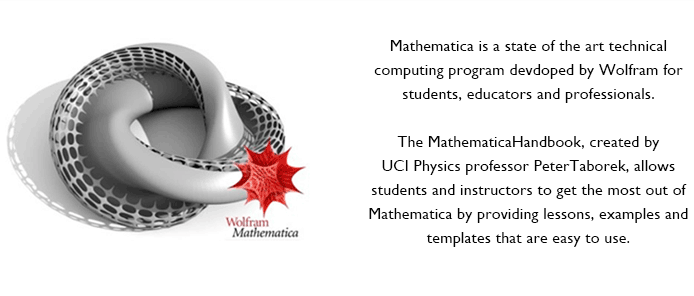The MathematicaHandbook is a download which is viewed as a notebook within your copy of Mathematica®. It provides lessons, examples and templates at your fingertips for a wide variety of problems in physics, chemistry, and engineering. It can be used as a supplementary text in any undergraduate or graduate level mathematical methods course which includes an introduction to modern mathematical software.
The MathematicaHandbook includes:

1) a Mathematica tutorial which provides a fast-paced but thorough introduction of basic syntax suitable for beginners. It also provides a discussion of more advanced topics such as programming with multi-line functions, symbolic processing with patterns, and importing and exporting data files, all illustrated with many “live” examples that can be executed within the notebook.

2) a comprehensive presentation of topics and problems encountered in a traditional mathematical methods course (linear algebra, vector analysis, ordinary and partial differential equations, boundary value problems, etc.) with a careful comparison of traditional versus Mathematica-based techniques for solving these types of problems.

3) Case studies and Applications which explores more advanced applications of Mathematica to  quantum mechanics, fluid mechanics, electricity & magnetism, etc.